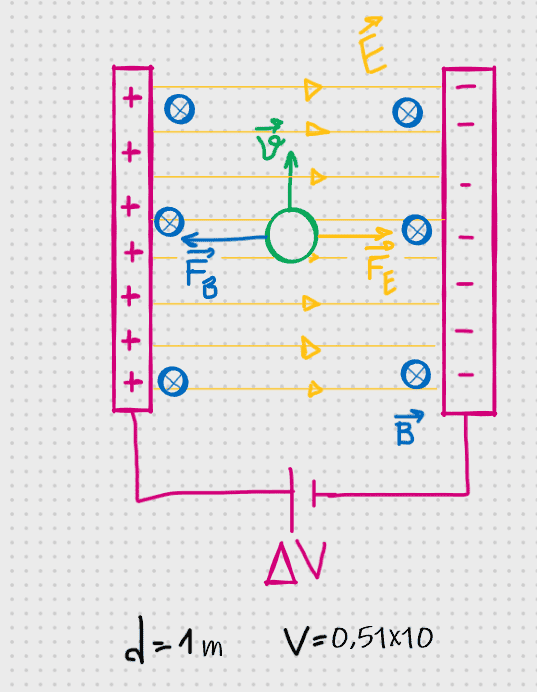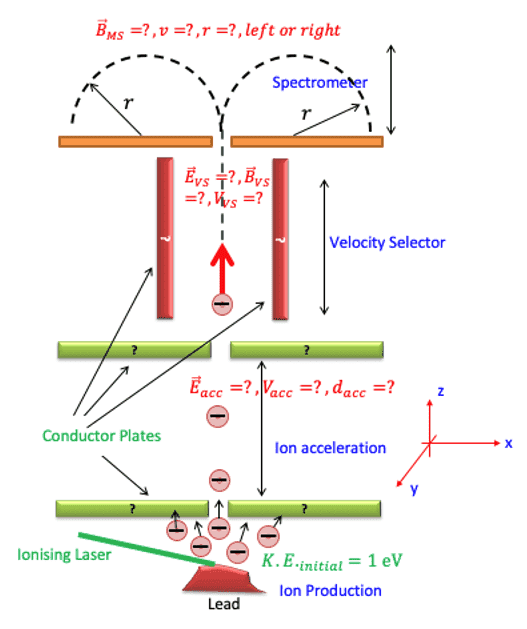# How the power of the voltage supply can be calculated?

• requied

#### requied

Homework Statement
What should be the power of the voltage supply below that we use for producing electric field .
Relevant Equations
P=IV = IR^2 =V^2/R
I know only these relevant equations for power. But here there is a different situation, we have a parallel plates which we don't have to know I current. How should I think of about this? I wonder if it can be thought of a capasitor or something?Assume that also we know E, B and velocity .

•Delta2

Did you give the complete statement of the problem? In your drawing you show a magnetic field and a particle moving through the E and B fields. But, the problem statement doesn't mention anything about these.

Are you supposed to find the power of the voltage supply or are you supposed to find the voltage of the power supply?

From what you have shown, it looks to be a simple capacitor, so no current is flowing. If I=0, then what is the power?

I am guessing that the OP is troubled by the following fact:
As the particle accelerates under the influence of electric field, if this accelerated movement corresponds to some sort of current, and where the energy comes from that increases the kinetic energy of the particle.

My answer to this is that though this movement is indeed a current, still this current is not drawn from the voltage source, and the energy that increases the kinetic energy of the particle does not come from the source but from the initial potential energy of the particle inside the field.

The energy to produce the E-field is drawn from the voltage source and it is $$E=\frac{1}{2}CV^2$$ where C the capacitance of the capacitor. This energy is drawn when capacitor is charged.

Last edited:
Relevant Equations:: P=IV = IR^2 =V^2/R

I know only these relevant equations for power.

You might want to verify those for future use, one of them is incorrect.

Are you supposed to find the power of the voltage supply or are you supposed to find the voltage of the power supply?
I spesified it on the homework statement.

If I=0, then what is the power?
It's that easy really? I supposed that I would do some complex calculations :/

As the particle accelerates under the influence of electric field, if this accelerated movement corresponds to some sort of current, and where the energy comes from that increases the kinetic energy of the particle.
I didn't ask the question for anything about the kinetic energy of the particle. You misunderstood me . I just wondered that what is the voltage supply's power.

It's that easy really? I supposed that I would do some complex calculations :/
I didn't ask the question for anything about the kinetic energy of the particle. You misunderstood me . I just wondered that what is the voltage supply's power.
No power is required to maintain the electric field in isolation. The moving particle, which you did not mention in the problem description, must be relevant.
Would I be right in thinking there is a stream of such particles? Whatever, please post the entire question as given to you.

This looks suspiciously like the sort of problem where one should calculate the potential difference between the deflection plates that will result in a linear trajectory for a given charged particle passing through a fixed magnetic field.

The OP needs to provide his definition of "power" for the voltage source, and the specifics of the particle.

please post the entire question as given to you.
This is a piece of a spectrometer question and this region above is the velocity selector region. The question says "What should be the power of the voltage supply that we will use in velocity selector region?".
I think it is not a problem to just take this area about the question. I assumed that we already know the values I found in other regionsthat will result in a linear trajectory for a given charged particle passing through a fixed magnetic field.
That will occur on spectrometer

•Delta2